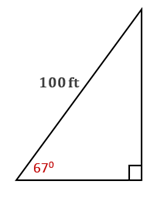Chapter 11.CT, Problem 11CT### Elementary Geometry for College St...

6th Edition
Daniel C. Alexander + 1 other
ISBN: 9781285195698

#### Solutions

Chapter
Section### Elementary Geometry for College St...

6th Edition
Daniel C. Alexander + 1 other
ISBN: 9781285195698
Textbook Problem
1 views

# A kite is flying at an angle of elevation of 670 with theground. If 100 ft of string have been paid out to thekite, how far is the kite above the ground? Answer to the nearest foot._________To determine

To find:

The height of the kite from the ground to the nearest foot.

Explanation

Given:

A kite is flying at an angle of elevation of 670 with the ground. If 100 ft of string have been paid out to the kite.

Approach:

In a right triangle, the sine ratio for an acute angle is the ratio oppsite sidehypotenuse.

Calculation:

From the diagram, hypotenuse = 100 ft, and the angle is 670.

Let the opposite side be x ft

### Still sussing out bartleby?

Check out a sample textbook solution.

See a sample solution

#### The Solution to Your Study Problems

Bartleby provides explanations to thousands of textbook problems written by our experts, many with advanced degrees!

Get Started

#### Find the area between the curves in Problems 19-22. 21.

Mathematical Applications for the Management, Life, and Social Sciences

#### Justify (3) for the case h 0.

Single Variable Calculus: Early Transcendentals, Volume I

#### For y = sin2 x + cos2 x, y = _____. a) 2 sin x 2 cos x b) 2 sin x cos x c) 4 sin x cos x d) 0

Study Guide for Stewart's Single Variable Calculus: Early Transcendentals, 8th